Refer to our Texas Go Math Grade 2 Answer Key Pdf to score good marks in the exams. Test yourself by practicing the problems from Texas Go Math Grade 2 Lesson 6.1 Answer Key Break Apart Ones to Add.

Explore

Use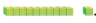Draw to show what you did.

FOR THE TEACHER • Read the following problem. Have children use blocks to solve. Griffin read 27 books about animals and 6 books about space, How many books did he read?
27 + 6 =
27 + 3 + 3
30 + 3 = 33
Explanation:
6 is written as 3 + 3
The ones are converted to tens
1 ten = 10 ones

Math Talk
Mathematical Processes

Describe what you did with the blocks.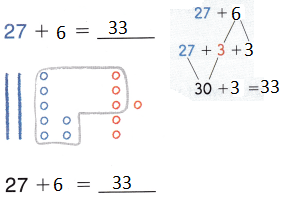Explanation:
6 is written as 3 + 3
The ones are converted to tens
1 ten = 10 ones

Model and Draw

Break apart ones to make a ten. Use this as a way to add.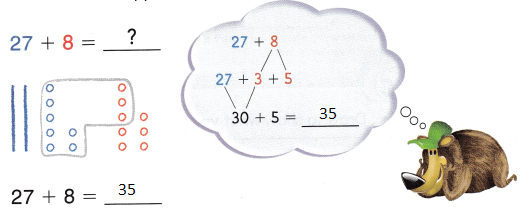27 + 3 + 5
30 + 5 = 35
Explanation:
8 is written as 3 + 5
The ones are converted to tens
1 ten = 10 ones

Share and Show

Draw quick pictures. Break apart ones to make a ten. Then add and write the sum.

Question 1.
15 + 7 = ____________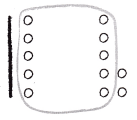15 + 7
15 + 5 + 2
20 + 2 = 22
Explanation:
7 is written as 5 + 2
The ones are converted to tens
1 ten = 10 ones

Question 2.
5 + 26 = ____________5 + 26
1 + 4 + 26
1 + 30 = 31
Explanation:
5 + 26 = 31
5 is written as 1 + 4
The ones are converted to tens
1 ten = 10 ones

Question 3.
37 + 8 = ____________37 + 8
37 + 3 + 5
40 + 5 = 45
Explanation:
37 + 8 = 45
8 is written as 3 + 5
The ones are converted to tens
1 ten = 10 ones

Question 4.
28 + 6 = ____________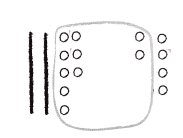28 + 6
28 + 2 + 4
30 + 4 = 34
Explanation:
28 + 6 = 34
6 is written as 2 + 4
The ones are converted to tens
1 ten = 10 ones

Problem Solving

Break apart ones to make a ten. Then add and write the sum.

Question 5.
23 + 9 = ____________
23 + 9
23 + 7 + 2
30 + 2 = 32
Explanation:
23 + 9 = 32
9 is written as 7 + 2
The ones are converted to tens
1 ten = 10 ones

Question 6.
48 + 5 = ____________
48 + 5
48 + 2 + 3
50 + 3 = 53
Explanation:
48 + 5  = 53
5 is written as 2 + 3
The ones are converted to tens
1 ten = 10 ones

Question 7.
7 + 58 = ____________
7 + 58
5 + 2 + 58
5 + 60 = 65
Explanation:
7 + 58 = 65
7 is written as 5 + 2
The ones are converted to tens
1 ten = 10 ones

Question 8.
33 + 9 = ____________
33 + 9
33 + 7 + 2
40 + 2 = 42
Explanation:
33 + 9 = 42
9 is written as 7 + 2
The ones are converted to tens
1 ten = 10 ones

Question 9.
6 + 27 = ____________
6 + 27
3 +  3 + 27
3 + 30 = 33
Explanation:
6 + 27 = 33
6 is written as 3 + 3
The ones are converted to tens
1 ten = 10 ones

Question 10.
49 + 4 = ____________
49 + 4
49 + 1 + 3
50 + 3 = 53
Explanation:
49 + 4 = 53
4 is written as 1 + 3
The ones are converted to tens
1 ten = 10 ones

Solve. Write or draw to explain.

Question 11.
H.O.T. Multi-Step Bruce sees 29 oak trees and 4 maple trees at the park. Then he sees double the number of pine trees as maple trees. How many trees does Bruce see?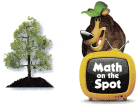________ trees
37 trees
Explanation:
Bruce sees 29 oak trees and 4 maple trees at the park.
Then he sees double the number of pine trees as maple trees.
4 + 4  = 8
29 + 8
29 + 1 + 7
30 + 7 = 37
37  trees that Bruce see

Question 12.
H.O.T. Jamal has a box with some toy cars in it He puts 3 more toy cars into the box. Now there are 22 toy cars in the box. How many toy cars were in the box before?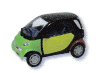_________ toy cars
19 toy cars
Explanation:
Jamal has a box with some toy cars in it He puts 3 more toy cars into the box.
Now there are 22 toy cars in the box.
22 – 3 = 19
19 toy cars were in the box before

Question 13.
Connect Devon saw 19 adults wearing hats and 3 children wearing hats. How many people did Devon see wearing hats?
(A) 22
(B) 21
(C) 23
Explanation:
Devon saw 19 adults wearing hats
and 3 children wearing hats.
19 +3
19 + 1 + 2
20+ 2 = 22
22 people that Devon see wearing hats

Question 14.
Draw quick pictures to show how to break apart ones to make a ten. Then find the sum.
27 + 8 = __________27 + 8 =
27 + 3 + 5
30 + 5 =35
Explanation:
27 + 8 = 35
8 is written as 3 + 5
The ones are converted to tens
1 ten = 10 ones

Question 15.
TEXAS Test Prep Alicia puts 25 books on the shelf. Trenton puts 8 books on the shelf. How many books do they put on the shelf?(A) 28
(B) 23
(C) 33
Explanation:
Alicia puts 25 books on the shelf.
Trenton puts 8 books on the shelf.
25 + 8
25 + 5 + 3
30 + 3 = 33
33 books that they put on the shelf

TAKE HOME ACTIVITY • Say a number from 0 to 9. Have your child name a number to add to yours to have a sum of 10.